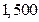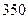Chapter 28, Problem 44P### Principles of Economics 2e

2nd Edition
Steven A. Greenlaw; David Shapiro
ISBN: 9781947172364

#### Solutions

Chapter
Section### Principles of Economics 2e

2nd Edition
Steven A. Greenlaw; David Shapiro
ISBN: 9781947172364
Textbook Problem

# If GDP now falls back toand the money supply falls towhat is velocity?

To determine

The velocity of money, given that the nominal GDP falls back to $1500 and money supply decreases to$350.

Explanation

The velocity of money is pace of circulation of money in the economy. The formula for calculating velocity of money is given below.

velocityofmoney=Nominal GDPMoneySupplyv=YMS

Given Information:

Money supply, MS= $350 Nominal GDP =$1500

### Still sussing out bartleby?

Check out a sample textbook solution.

See a sample solution

#### The Solution to Your Study Problems

Bartleby provides explanations to thousands of textbook problems written by our experts, many with advanced degrees!

Get Started

#### Find more solutions based on key concepts